# Algebraic analysis

Jump to: navigation, search

A phrase initially used by J.P. Lagrange in 1797 in the title of his book [a1] to indicate that most of the results have been obtained by algebraic operations on analytic quantities. In that general and common sense, this name, adopted also by A.L. Cauchy, was used in the 19th century and in the 20th century (cf. [a2], [a3], [a5]; see also Microlocal analysis).

The main idea of algebraic analysis in its present (1996), more strict, sense derives from the fact that the differential operatoris right-invertible in several function spaces.

The foundations of algebraic analysis are as follows. Let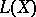be the set of all linear operators (cf. Linear operator) with domains and ranges in a linear space(in general, without any topology) over a fieldof scalars of characteristic zero and let. Let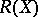be the set of all right-invertible operators inand let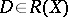. Let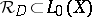be the set of all right inverses for, i.e.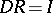(the identity operator) if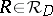. Moreover,. For all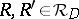,,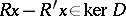, i.e. the difference of two primitives is a constant. Let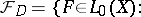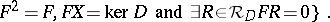Any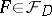is said to be an initial operator for(corresponding to an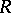). One can prove that any projection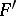ontois an initial operator forcorresponding to a right inverse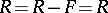independent of the choice of an. If two right inverses (respectively, initial operators) commute, then they are equal. Thus this theory is essentially non-commutative. An operator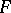is initial forif and only if there is ansuch that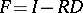on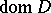. The last formula yields (by a two-line induction) a Taylor formula:From these facts one can obtain a calculus and solutions to linear equations (under appropriate assumptions on solving equations). If the field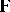is algebraically closed (cf. Algebraically closed field), then solutions of linear equations with scalar coefficients can be calculated by a decomposition of a rational function into vulgar fractions (similarly as in operational calculus). Ifis a commutative algebra,andsatisfies the Leibniz conditionfor, then the trigonometric identity holds. Some results can be proved also for left-invertible operators, even for operators having either finite nullity or finite deficiency. There is a rich theory of shifts and periodic problems. Recently, logarithms and anti-logarithms have been introduced and studied (even in non-commutative algebras). This means that algebraic analysis is no longer purely linear.

The main advantages of algebraic analysis are:

simplifications of proofs due to an algebraic description of the problems under consideration;

algorithms for solving "similar" problems, although these similarities could be rather far each from another and very formal;

several new results even for the classical operator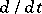(which is, indeed, unexpected).

There are several applications to ordinary and partial differential equations with scalar and variable coefficients, functional-differential equations and for discrete analogues of these equations, for instance, for difference equations. There are also some results for non-linear equations.

It should be pointed out that in algebraic analysis a notion of convolution of functions is not necessary. Also, there is no need to have a field structure. This, together with the non-commutativity of right inverses and initial operators, shows the essential distinction between algebraic analysis and operational calculus.